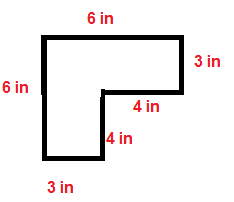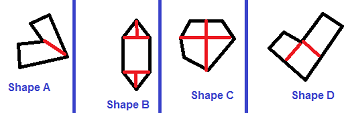# Finding the Area of an Irregular Hexagon

Instructions:

question 1 of 3

### Find the area of this irregular hexagon.Create Your Account To Take This Quiz

As a member, you'll also get unlimited access to over 79,000 lessons in math, English, science, history, and more. Plus, get practice tests, quizzes, and personalized coaching to help you succeed.

Try it risk-free for 30 days. Cancel anytime

### 2. Which one of the following irregular hexagons is correctly divided into rectangles and triangles so that the area can be calculated?Create your account to access this entire worksheet
Quizzes, practice exams & worksheets
Certificate of Completion
Create an account to get started

There are many rules to remember about working with different shapes. This quiz will tell how well you remember the steps to find the area of an irregular hexagon. Questions on the quiz will ask you to define an irregular hexagon, point out its features, and find the area of sample irregular hexagons.

## Quiz & Worksheet Goals

You can point out what you remember about:

• Measuring the area of irregular hexagons
• Describing an irregular hexagon
• Dividing these shapes into triangles and rectangles
• Deciding what info is needed for finding the area

## Skills Practiced

• Critical thinking - look at information about irregular hexagons in a different way
• Problem solving - use what you learned to solve irregular hexagon area practice problems
• Knowledge application - use what you know to answer questions about what is needed to find the area of an irregular hexagon

Go over further information about this topic by reading the connected lesson, Finding the Area of an Irregular Hexagon. Use the lesson to decide on answers for the quiz questions, but also look over the following:

• The definition of area and related formulas
• 3 steps for finding the area
• Irregular hexagon examples
Final ExamMath for Kids
Status: Not Started
Chapter ExamGeometry for Elementary School
Status: Not Started

Support